Next: Patriot Missile Software Up: No Title Previous: Sources of Error

# Floating Point Number System

A floating point number system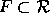is a subset of the real numbers whose elements have the form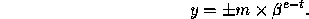The system F is characterized by four integer parameters:

• the base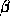(sometimes called the radix),
• the precision t, and
• the exponent range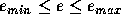.

The mantissa m is an integer satisfying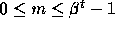. To ensure a unique representation for each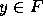, it is assumed that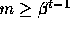if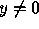, so that the system is normalized. In other words the first digit of the mantissa is non-zero. The range of the non-zero floating point numbers in F is given by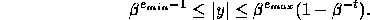It follows that every real number x lying in the range of F can be approximated by an element of F with a relative error no larger than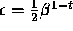. The quantity is called the machine epsilon or unit roundoff. It is the most useful quantity associated with F and is ubiquitous in the world of rounding error analysis.

We are mainly interested in IEEE floating point number system. Over the last few years it has become a standard.

IEEE Single Precision Arithmetic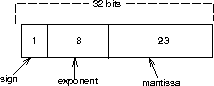Figure 1: IEEE Single Precision Arithmetic

Based on these values the various parameters are: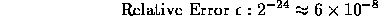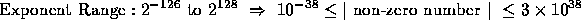IEEE Double Precision Arithmetic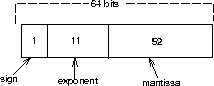Figure 2: IEEE Double Precision Arithmetic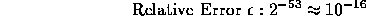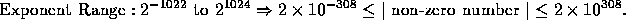Roundoff error results since the true value of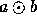, where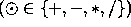can't be represented exactly and needs to be rounded off. If we roundoff as accurate as possible, and the floating point result is within the exponent range than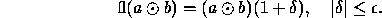We say that fl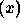overflows if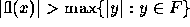and underflows if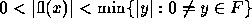. To see the impact of rounding and truncation, lets consider the following C program.

```#include <stdio.h>
#include <math.h>

main()
{

float f;
double d,p;
int i;

i = 32768 * 32768 + 256 + 128 + 64 + 32 + 16 + 8 + 4 + 2 + 1;
f = (float) i;
d = (double) i;

p = fabs(f - i)/i;

printf("%d  %4.16f %4.16lf %4.16f \n",i,f,d,p);

}
```
What are the values of i, f, d and p? The errors caused to improper rounding or truncation can be very serious at times.Next: Patriot Missile Software Up: No Title Previous: Sources of Error

Dinesh Manocha
Wed Jan 8 00:43:08 EST 1997